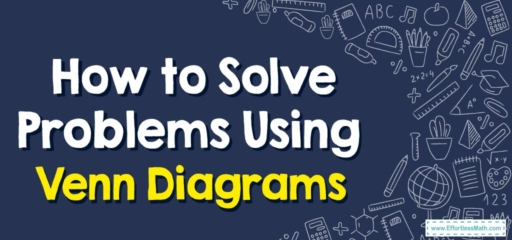# How to Solve Problems Using Venn Diagrams

Venn diagrams are visual tools often used to organize and understand sets and the relationships between them. They're named after John Venn, a British philosopher, and logician who introduced them in the 1880s. Venn diagrams are frequently used in various fields, including mathematics, statistics, logic, computer science, etc. They're handy for solving problems involving sets and subsets, intersections, unions, and complements.## A Step-by-step Guide to Solving Problems Using Venn Diagrams

Here’s a step-by-step guide on how to solve problems using Venn diagrams:

### Step 1: Understand the Problem

As with any problem-solving method, the first step is to understand the problem. What sets are involved? How are they related? What are you being asked to find?

### Step 2: Draw the Diagram

Draw a rectangle to represent the universal set, which includes all possible elements. Each set within the universal set is represented by a circle. If there are two sets, draw two overlapping circles. If there are three sets, draw three overlapping circles, and so forth. Each section in the overlapping circles represents different intersections of the sets.

### Step 3: Label the Diagram

Each circle (set) should be labeled appropriately. If you’re dealing with sets of different types of fruits, for example, one might be labeled “Apples” and another “Oranges”.

### Step 4: Fill in the Values

Start filling in the values from the innermost part of the diagram (where all sets overlap) to the outer parts. This helps to avoid double-counting elements that belong to more than one set. Information provided in the problem usually tells you how many elements are in each set or section.

### Step 5: Solve the Problem

Now, you can use the diagram to answer the question. This might involve counting the number of elements in a particular set or section of the diagram, or it might involve noticing patterns or relationships between the sets.

Make sure your answer makes sense in the context of the problem and that you’ve accounted for all elements in the diagram.

### What people say about "How to Solve Problems Using Venn Diagrams - Effortless Math: We Help Students Learn to LOVE Mathematics"?

No one replied yet.

X
30% OFF

Limited time only!

Save Over 30%

SAVE $5 It was$16.99 now it is \$11.99